# One third 2

One third of all students in class live in a house. If here are 42 students in a class, how many of them live in house?

h =  14

### Step-by-step explanation: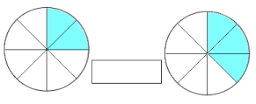Did you find an error or inaccuracy? Feel free to write us. Thank you!Tips to related online calculators
Need help to calculate sum, simplify or multiply fractions? Try our fraction calculator.

## Related math problems and questions:

• There 12There are 42 students in the class and 2/3 of them are girls. How may girls are there in the class?
• Pupils 7There are 40 pupils in a certain class. 3/5 of the class are boys. How many are girls?
• English and math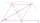1/5 of a class study English, and 3/5 study mathematics. If the class consist of 80 students, how many students study English and mathematics in the class?
• Math test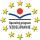In class 6.A are 26 students, and the teacher had managed to give tests to 13 students. 13 testes he gave in 6.5 min. How many pupils still haven't test? How long will it take the teacher to give tests to 43 students?
• FortyForty five of the 80 students were girls. What is the ratio of girls to boys?
• ShirtsIn a classroom, 1/6 of the students are wearing blue shirts and 2/3 are wearing white shirts. There are 36 students in the class. How many students are wearing a shirt other than blue or white?
• Class 8.AThree quarters of class 8.A went skiing. Of those who remained at home one third was ill and the remaining six were on math olympic. How many students have class 8.A?
• Boys and glasses2/3 of the students in Sarah’s class are boys. Of the boys, 1/3  of them wear glasses. What fraction of the students wear glasses?
• Students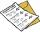The class has 22 students. 2 of which have assessments of 2. The count of assessment of 1 is three times more than the count of students with an assessment of 3. How many students have an assessment of 1, and how many students have an assessment of 1.
• Report 2A School reports students to teacher ratio of 6:1. If there are 45 teachers in the School, how many students are there?
• Fractions and mixed numerals(a) Convert the following mixed numbers to improper fractions. i. 3 5/8 ii. 7 7/6 (b) Convert the following improper fraction to a mixed number. i. 13/4 ii. 78/5 (c) Simplify these fractions to their lowest terms. i. 36/42 ii. 27/45 2. evaluate the follow
• A teacher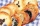A teacher baked 5 dozen cookies for the 16 students in her class. If the teacher divided the cookies equally among her students, how many cookies did she give each student?
• School trip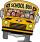School trip cost 247.2 Eur for one class (24 students). How much would a trip cost for two classes? (both classes together have 53 students)
• Soup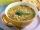47% of pupils at the school to eat in the canteen, 1/47 of students who eat in the canteen eat lunch soup. a) What percentage of pupils who eat in the canteen and eat soup for lunch? b) What percentage of all pupils of school eat lunch soup?
• Seating rules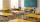In a class are 24 seats but in 7.B class are only 18 students. How many ways can students sit? (The class has 12 benches. A bench is for a pair of students.) Result (large number) logarithm and thus write down as powers of 10.
• TimeWrite time in minutes rounded to one decimal place: 3 h 48 m 45 s.
• Lunch timeIn a cafeteria, 3/10 of the students are eating salads, and 3/5 are eating sandwiches. There are 30 students in the cafeteria. How many students are eating lunches other than salads or sandwiches?